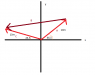# Vectors: Finding Magnitude and Angle

• tensirk

## Homework Statement

In the sum A+B = C, vector A has a magnitude of 10.0 m and is angled 46.5° counterclockwise from the +x direction, and vector C has a magnitude of 15.0 m and is angled 23.5° counterclockwise from the -x direction. What are the magnitude and the angle (relative to +x) of B?
________ m at _______ ° counterclockwise from the +x direction

a2+b2=c2

## The Attempt at a Solution

I have tried this:
A: (10cos(46.5))= 6.88; (10sin(46.25))= 7.25
C: (15cos(23.5))= 13.76; (15sin(23.5))= 5.98

Then:
13.76-6.88= 6.88
5.98-7.25=-1.27

After this I used the Pythagorean Theorem...

√(-1.27)2+(6.88)2 = 7m

From there I calculated tan-1(-1.27)/(6.88)= -10.46 degrees

Also obviously incorrect.

Help is greatly appreciated!

Did you try sketching the vectors first?

Yes, but I am kind of unsure as to what "counterclockwise from the (-)(+)x direction" is referring to.

#### Attachments

•vectors.png
2.2 KB · Views: 439
tensirk:

Your C vector is laid out incorrectly. Its angle is measured CCW from the negative x-axis
(remember, clockwise is always the same direction from the observer's viewpoint.)